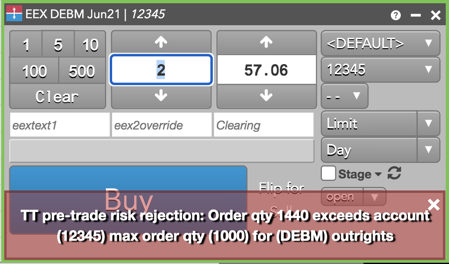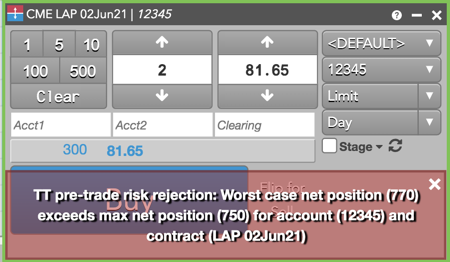Risk Limits

# Risk calculations for trading in flow

TT calculates position limits for energy products in either flow or contracts based on the "Enforce energy limits in Contracts" setting in Setup. When this option is unchecked for an energy product, TT calculates risk in flow.

When calculating risk limits for energy products, TT uses the following delivery values from the instrument specification:

• roundLotQty — The minimum quantity allowed for trading one contract.
• numberOfBlocks — The number of delivery periods.
• minLotSize — The amount to be delivered in each delivery period.

Note: The instrument specification can be shown in the TT workspace by selecting a contract and clicking Ctrl-Shift-X on your keyboard.

The following table shows examples of how risk is calculated per exchange for trading energy products in flow and contracts using the Trade application on TT.

Exchange Contract Delivery Values Submitted Order Quantity Risk Check in TT Displayed Order Quantity
CME LAP 02Jun21 roundLotQty=1
numberOfBlocks=35
minLotSize=50

qty = 1

Flow: qty * numberOfBlocks = 1*35

Contracts: qty/roundLotQty = 1/1

Flow: qty * minLotSize = 1*50 = 50

Contracts: qty = 1

EEX DEBM Jun21 roundLotQty=1
numberOfBlocks=720
minLotSize=720

qty = 1

Flow: qty * numberOfBlocks = 1*720

Contracts: qty/roundLotQty = 1/1

Flow: qty * minLotSize = 1*720 = 720

Contracts: qty = 1

ICE PJM S May20 roundLotQty=50
numberOfBlocks=352
delivery unit=1 (ICE does not consider minLotSize)

qty = roundLotQty * order quantity (1) * delivery unit = 50

Flow: qty * numberOfBlocks = 50*352

Contracts: qty/roundLotQty = 50/50

Flow: qty = 50

Contracts: qty/roundLotQty = 50/50 = 1

IDEM IDEPY roundLotQty=1
numberOfBlocks=1
minLotSize=8760

qty = 1

Flow: qty * numberOfBlocks = 1*1

Contracts: qty/roundLotQty = 1/1

Flow: qty * minLotSize = 1*8760 = 8760

Contracts: qty = 1

Nodal AAG roundLotQty=1
numberOfBlocks=704>
minLotSize=704

qty = 1

Flow: qty * numberOfBlocks = 1*704

Contracts: qty/roundLotQty = 1/1

Flow: qty * minLotSize = 1x704 = 704

Contracts: qty = 1

## Position Limit Example: EEX Energies risk in flow

For the DEBM Jun21 contract on EEX, the following account position limits are set:

• Max Order Quantity (Outrights) — 1000
• Max long position per contract — 7500
• Enforce energy limits in Contracts checkbox — Unchecked (risk is calculated in flow)

Question: If the trader attempts to buy 2 DEBM Jun21 contracts using this account, will the order be accepted or rejected?

Answer: The order is rejected. Risk is calculated in flow for the DEBM Jun21 contract on EEX: order quantity * numberOfBlocks = 2*720. The quantity of 2 contracts in DEBM Jun21 is equivalent to 1440 lots, which exceeds the Max Order Quantity of 1000 in Setup. The trader receives the following error message in their TT workspace.## Position Limit Example: CME Energies risk in flow

For the LAP 02Jun21 contract on CME, the following account position limits are set:

• Max Order Quantity (Outrights) — 100
• Max long position per contract — 750
• Enforce energy limits in Contracts checkbox — Unchecked (risk is calculated in flow)
• Current position = 700

Question: If the trader attempts to buy 2 LAP 02Jun21 contracts using this account, will the order be accepted or rejected?

Answer: The order is rejected. Although the "Max order quantity" passes the risk check (qty * numberOfBlocks = 2 * 35 = 70 < 100), the resulting position in LAP 02Jun21 ((20 * 35) + (2 * 35) = 770) exceeds the position limit of 750 for the LAP contract. The trader receives the following error message in their TT workspace.